# Heat and Entropy Lines Visualization of Natural Convection Between Hot Inner Circular Cylinder and Cold Outer Sinusoidal Cylinder

Heat and Entropy Lines Visualization of Natural Convection Between Hot Inner Circular Cylinder and Cold Outer Sinusoidal Cylinder

Mohammed Y. Jabbar Saba Y. Ahmed Hameed K. Hamzah Farooq H. Ali

Department of Mechanical Engineering, College of Engineering, University of Babylon, Babylon 51001, Iraq

Corresponding Author Email:
eng.farooq.hassan@uobabylon.edu
Page:
1151-1162
|
DOI:
https://doi.org/10.18280/ijht.370425
20 April 2019
|
Accepted:
16 December 2019
|
Published:
26 December 2019
| Citation

OPEN ACCESS

Abstract:

It is necessary for the heat transfer problems to provide information about the critical parameters that affect the efficiency to improve the status of the system. Major part of these information can be obtained by the investigations of the heatlines and entropy generation. Therefore, the laminar free convection in an enclosure forming by a hot inner circular cylinder and cold sinusoidal outer cylinder at different amplitude (λ=1, 2, and 4) and Rayleigh Number range (103-106) is numerically studied. Heatlines, streamlines, isotherms, and entropy generation are obtained using finite element technique based on the Galerkin method. The inner and outer cylinders are assumed to be kept at a constant temperature. Air is the   working fluid with Prandtl number (0.71). The results showed that the fluid circulations are governed by two combined facts; the first one is the buoyancy force that excited by the increasing of Rayleigh number producing bigger circulations and the second is the increasing in the nondimensional amplitudes which damps the flow.

Keywords:

natural convection, heatlines, entropy generation, sinusoidal corrugated cylinder, differential heated enclosure

1. Introduction

In recent ten years, the wavy or corrugated enclosures walls are wide spread for various engineering applications, such as cooling of the electronic device, heat exchanger, air cooling systems, and solar heating process. Thus, people are looking for introducing a useful way to enhance the rate of heat transfer. In addition, the geometry of the enclosure wall and the amplitude have a significant effect on the temperature distribution and fluid flow.

The sinusoidal term was appeared in the literature by two meanings; in the first one, the enclosure walls are curvilinear and formed as sinusoidal surfaces, while in the second one the governing equations of the boundary conditions distributions are assumed to be sinusoidal.

A lot of researches have been accomplished for investigation heat transfer and fluid flow inside enclosures. Sheikholeslami and Rokni  studied effect of the electrical filed on the viscosity of a nanofluid inside cavity of a sinusoidal wall. The results showed that the electrical field enhanced the convective heat transfer. Sheikholeslami and Rokni  studied heat transfer and fluid flow in a porous complex-shaped enclosure containing Cu-O water nanofluid subjected to a magnetohydrodynamic field. The results showed that the augmentation of Hartman number led to diminish the temperature gradient. Additionally, Sheikholeslami et al. [3-9] presented extensive works regarding heat transfer and fluid flow inside enclosure of different shapes and configurations and different types of working fluids. Sheikholeslami and Shehzad  presented the translation behavior of a nanofluid in porous media. The results described that the generated isotherms tended to be more complicated with the escalation of the buoyancy force. Sheikholeslami and Shamlooei  simulated influence of a magnetic source on a Fe3O4-water nanofluid as passes through a porous medium enclosure. The results presented that the velocity of nanofluid and Nusselt number decreased with the increasing in Hartman Number. Sheikholeslami and Ozotop  accomplished similar study to what was done in his previous study , but they replaced the half-cylinder of the outer curvilinear wall enclosure by a quarter one. Same boundary conditions were applied. Sheikholeslami and Ganji  performed similar analysis as that presented in paper , but they used CuO instead of Fe3O4 and studied how the magnetic nanofluid disposal changed. Sheikholeslami et al , investigated numerically the natural convection between a cold outer cylinder enclosure a hot inner curvilinear-wall cylinder by finite element method. Sheikholeslami and Ellahi  investigated effect of the electrical field of Fe3O4 nanofluid in an enclosure. The lower wall of the enclosure was permitted to slide horizontally, while the upper wall was kept fixed and has a sinusoidal form. It was conducted that the supplied voltage could change the streamlines. Sheikholeslami et al  accomplished an investigation for the magnetic field effect on natural convection inside curved shape enclosure. The results depicted that the Nusselt number decreases with increasing Hartman number.

Tang et al.  proposed a new study for a two-dimensional quarter-circular enclosure sinusoidal inner and outer walls. It was concluded that the Nusselt number increases dramatically with the nanofluid volume fraction. Sherement et al.  performed a numerical study on the unsteady natural convection of wavy wall enclosure containing a nanofluid subjected to a magnetic field. Yousaf and Usama  presented a numerical study of natural convection in a square enclosure with the existence of roughness elements on the vertical walls. Results explained that the wall roughness affected the streamlines and the isotherms. Sheremet and Pop  studied numerically the natural convection of a nanofluid inside a porous wavy wall enclosure with sinusoidal boundary conditions on both sides. It was found that the Nusselt number increases with Rayleigh number. Nabavizadeh et al.  used Lattice Boltzmann method to investigate the natural convection of a hot square enclosure containing a sinusoidal cylinder. The results showed some variations in Nusselt number due to the increasing in amplitude and undulation numbers. Oztop et al. [15, 16], numerically studied the mixed and natural convection of a wavy walled enclosure with various boundary conditions. Sompong and Witayangkurm  simulated natural convection in a two-waved vertical wall enclosure filled with porous media. Results showed a decrease in density with a decrease in the number of Darcy and Raleigh. A numerical study on mixed convection lid-driven wavy wall and heated central square solid body enclosure exposed to a magnetic field was performed by Nasrin . The results showed that magnetic field removal registered a maximum heat transfer rate for the highest Prandtl number. Khanafer et al.  implemented a numerical investigation on streamlines and isotherms behavior in a sinusoidal vertical wavy-wall enclosure filled with a porous medium. It was illustrated that number of undulation and the amplitude of the sinusoidal surface changed the heat transfer characteristics inside the enclosure. The natural convection within the enclosure with two vertical, isothermal and sinusoidal walls and two isolated, straight and horizontal walls was numerically predicted by Mahmud et al. . Kim et al.  conducted a numerical study of natural convection due to the difference in temperature between the cold wall of the hollow square enclosure and the hot circle cylinder inside it. The results showed that the location of the inner circular cylinder along the vertical center line affected both fluid flow and heat transfer inside the enclosure. Hussein  presented a numerical study for to analyze heat lines and entropy generation in a sinusoidal side wall-inclined porous enclosure subjected to a magnetic field. The results explained that the horizontal magnetic field enhances the entropy generation more than that corresponding in the vertical direction. Matrod et al.  studied free convection inside the annulus gap which filled with air. Triangular fins were attached to the hot inner circular cylinder to enhance heat transfer inside the annulus gap. Al-Amir et al  presented a numerical study to show effect of wavy wall location of natural convection inside square enclosure having a concentric hot circular cylinder. The results showed that heat transfer was influenced by Rayleigh number and the number of undulations. Basak et al.  presented a numerical visualization of thermal transport within triangular cavities along with heatline analysis. It was observed that conduction heat transfer was the dominant at Ra=107. Natarajan et al.  studied numerically natural convection in various trapezoidal enclosures with various wall boundary conditions based on a comprehensive heatline approach. It was concluded that the Nusselt number was distributed sinusoidally at Prandtl number=1000. The entropy generation caused by natural convection within a square inclined differentially heated enclosure was numerically investigated by Shavic et al. . The results showed the fluid flow entropy generation showed an increase with an increase of inclination angle. Based on Lattice Boltzmann technique, Rahimi et al. [28-29] performed an empirical and experimental studies of entropy generation and heatline through rectangular and L-shaped enclosures filled by a nanofluid, respectively. Hussein et al.  used a numerical method to compute natural convection under unsteady state conditions and entropy generation in an inclined three-dimensional enclosure. It was noticed that at lower Rayleigh number, the enclosure inclination showed a negligible effect on the total entropy generation. Ghasemi and Siavashi  used a numerical method to simulate the entropy generation of a nanofluid in a porous enclosure under natural convection state and linear temperature distributions applied on the side walls. Lattice Boltzmann technique was used in the study of Alsabery et al.  to study entropy generation and natural convection of a concentric solid inside a square enclosure filled with nanofluid. It was indicated from the results that the heat transfer was controlled by the inner solid size and the thermal conductivity ratio. Finally, Abdulkadhim et al.  studied the natural convection inside trapezoidal enclosure having a solid baffle inside. Three different heights for the baffle were taken. Cu-Water nanofluid was chosen as a working fluid, entropy generation inside the enclosure has been studied as a result of fluid flow and heat transfer.

Other people accomplished their works in terms of sinusoidal boundary conditions instead of geometry. Roslan et al.  studied free convection heat transfer at time dependent sinusoidal boundary condition around inner hot circular cylinder fixed in the middle of a cold walls-square enclosure. It was noted that the maximum heat transfer rate occurred at the period between 25π and 30π. Bouhalleb and Abbassi  showed a numerical investigation of free convection within two-dimensional rectangular enclosure occupied by CuO water nanofluid. The two horizontal walls were perfectly insulated and the left vertical side was heated by a sinusoidal temperature distribution source. It was concluded that the system time response was affected by the temperature amplitude and frequency. Alsabery et al.  studied numerically effect of side sinusoidal heating and finite wall thickness on the convection‎ within a square porous enclosure. The work outputs showed that the heat transfer rate was strongly excited as the sinusoidal heating increased. Effect of the sinusoidal heating on the mixed convection inside a square enclosure, occupied by a porous medium was numerically analyzed by Zainuddin et al. . The results showed that the streamlines and isotherms were significantly affected as the sinusoidal heating shifted. Unsteady state mixed convection inside a square enclosure containing Ag-water nanofluid has been carried out by Karim et al. . The frequency of sinusoidal boundary condition applied to the bottom horizontal wall was assumed to vary in different values. It was noted that the positive and negative frequencies had a significant impact on the flow patterns. Dutta et al.  performed a numerical study of natural convection and entropy generation in a quadrant porous enclosure with a non-uniform heating from below. It was observed that at low values of Darcy number, the entropy generation due to heat transfer was important and governed the system. Finally, Laouer and Djeghiour  applied a sinusoidal boundary condition at the bottom horizontal wall and used the Lattic Boltzmann method for the numerical investigation of the magnetohydrodynamic natural convection within the enclosure.

According to the best experience of the authors, there is no a significant-reported study for the heat lines and entropy generation behavior has been done at various amplitudes of a wavy wall-cylinder enclosure. The existing problem of the present work is to examine the effect of the variation of amplitude (λ) on streamlines, isotherms, heat lines, and entropy generation at different Rayleigh numbers. Galerkin finite element (GFE) technique is carried out to solve the governing equations with different Rayleigh numbers and various amplitudes for the outer cylinder.

2. Physical Model Description

The schematic diagram of the present study is shown in Figure (1). The diagram shows an outer wavy cylinder kept at a cold temperature (Tc) and an inner circular cylinder kept at hot temperature (Th). The governing equations of the outer wavy cylinder are:

$X=\left[R_{o}+\lambda \sin \left(n * \varphi * \frac{\pi}{180}\right) / n\right] *\left(\cos \left(\varphi * \frac{\pi}{180}\right)\right)$ (1)

$Y=\left[R_{o}+\lambda \sin \left(n * \varphi * \frac{\pi}{180}\right) / n\right] *\left(\sin \left(\varphi * \frac{\pi}{180}\right)\right)$ (2)

where, Ro is the basic outer circle radius, λ is the non-dimensional amplitude, n is the number of corrugations and φ is the angle of rotation. The annular space between the two cylinders (Ro-Ri) is equal to unity where Ri=1. Air was chosen as a working fluid with Prandtl number is fixed at Pr=0.71. Air density is not influenced by pressure change (incompressible fluid). The temperature difference between the inner and outer cylinders Th>Tc, generates the natural convection due to the buoyancy force. All thermo-physical properties are assumed to be constant except density, which obeys to the Boussinesq approximation.

## 1.png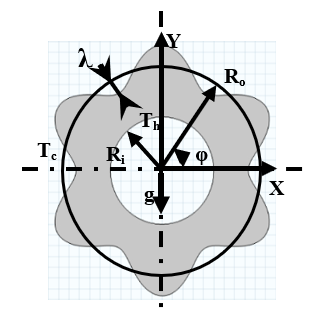Figure 1. Simplified diagram of physical of the present study, N=6

3. Governing Equations and Boundary Conditions

The present study assumes the flow is laminar. The analysis is accomplished in two dimensions only. The response state is steady. The viscous dissipation, heat generation, and radiation are neglected. The dimensional governing equations for the case study, continuity, momentum, and energy, can be written as:

$\frac{\partial u}{\partial x}+\frac{\partial v}{\partial y}=0$    (3)

$\rho\left(u \frac{\partial u}{\partial x}+v \frac{\partial u}{\partial y}=-\frac{\partial p}{\partial x}+\mu\left(\frac{\partial^{2} u}{\partial x^{2}}+\frac{\partial^{2} u}{\partial y^{2}}\right)\right.$     (4)

$\rho\left(u \frac{\partial v}{\partial x}+v \frac{\partial v}{\partial y}=-\frac{\partial p}{\partial y}+\mu\left(\frac{\partial^{2} v}{\partial x^{2}}+\frac{\partial^{2} v}{\partial y^{2}}\right)+\rho g \beta\left(T-T_{c}\right)\right.$     (5)

$\rho c_{p}\left(u \frac{\partial T}{\partial x}+v \frac{\partial T}{\partial y}=K\left(\frac{\partial^{2} T}{\partial x^{2}}+\frac{\partial^{2} T}{\partial y^{2}}\right)\right.$    (6)

where, u and v are the velocity components in the x and y-direction, respectively. T is the temperature of the fluid, p is the pressure. The other physical properties are described in the nomenclature. To transform the governing equation from dimensional form to dimensionless form, the following dimensionless variables are used and specified below:

$X=\frac{x}{R}, Y=\frac{y}{R}, U=\frac{u R}{\alpha}, V=\frac{v R}{\alpha}, P=$

$\frac{p R^{2}}{\mu \alpha}, \theta \frac{T-T_{c}}{T_{h}-T_{c}},$ where $R=R_{o}-R_{i}=1$    (7)

By assuming

\begin{aligned} \rho=1, \mu=1, K=& 1, \rho c_{p}=\operatorname{Pr} \operatorname{soc}_{p} \\ &=\operatorname{Pr} \text {and} \text {bod} y \text { force } F_{y}=G r * \theta \\ &=\frac{R a}{P r}\left(T-T_{c}\right) \end{aligned}

and using dimensionless variable in Eq. (7). Then, the Eqns. (3-6) can be transformed into the dimensionless form as follows:

$\frac{\partial U}{\partial X}+\frac{\partial V}{\partial Y}=0$     (8)

$U \frac{\partial U}{\partial X}+V \frac{\partial U}{\partial Y}=-\frac{\partial P}{\partial X}+\left(\frac{\partial^{2} U}{\partial X^{2}}+\frac{\partial^{2} U}{\partial Y^{2}}\right)$     (9)

$U \frac{\partial V}{\partial x}+V \frac{\partial V}{\partial y}=-\frac{\partial P}{\partial Y}+\left(\frac{\partial^{2} V}{\partial X^{2}}+\frac{\partial^{2} V}{\partial Y^{2}}\right)+R a P r \theta$      (10)

$\operatorname{Pr}\left(U \frac{\partial \theta}{\partial X}+V \frac{\partial \theta}{\partial Y}\right)=\left(\frac{\partial^{2} \theta}{\partial X^{2}}+\frac{\partial^{2} \theta}{\partial Y^{2}}\right)$    (11)

where, $R a=\frac{g \beta\left(T_{h}-T_{C}\right) R^{3}}{\alpha v}, \operatorname{Pr}=\frac{v}{\alpha}$

The flow inside the annular space is described by stream function, which estimated from velocity components U & V. The relation between velocity components and stream function for two-dimensional study are:

$U=\frac{\partial \Psi}{\partial Y}, V=-\frac{\partial \Psi}{\partial X} \& \frac{\partial^{2} \Psi}{\partial X^{2}}+\frac{\partial^{2} \Psi}{\partial Y^{2}}=\frac{\partial U}{\partial Y}-\frac{\partial V}{\partial X}$ (12)

The local and average Nusselt number is calculated from the following two expressions as:

$N u_{L}=\frac{\partial \theta}{\partial n}$ (13)

$N u_{a v e}=\frac{1}{2 \pi} \int_{0}^{2 \pi} N u_{L}(\varphi) d \varphi$ (14)

The heat transfer visualization is carried out using heat line expression. The heat line expression is intended using the mathematical formulation called heat function. The heat function formulation derived from the first derivative of the conduction equation ($-\frac{\partial \theta}{\partial X},-\frac{\partial \theta}{\partial Y}$) as well as convection equation ($U \theta, V \theta$). The heat function can express as follows:

$\frac{\partial \Pi}{\partial Y}=U \theta-\frac{\partial \theta}{\partial x}$ and $\frac{\partial \Pi}{\partial x}=-V \theta+\frac{\partial \theta}{\partial Y}$ (15)

The above equation can be written as follows:

$\frac{\partial^{2} \Pi}{\partial X^{2}}+\frac{\partial^{2} \Pi}{\partial Y^{2}}=\frac{\partial}{\partial Y}(U \theta)-\frac{\partial}{\partial X}(V \theta)$ (16)

The boundary conditions for the present study are given as follows:

Internal wall: $\theta=1, U=0, V=0$

External wall: $\theta=0, U=0, V=0$

For the matter under analysis, the non-dimensional local entropy generation is contributed by the entropy generation due to temperature gradients and entropy generation due to fluid friction can be written as:

$S_{L, H T}=\left(\frac{\partial \theta}{\partial X}\right)^{2}+\left(\frac{\partial \theta}{\partial Y}\right)^{2}$ (17)

$S_{L, F F}=\phi\left\{2\left[\left(\frac{\partial U}{\partial X}\right)^{2}+\left(\frac{\partial V}{\partial Y}\right)^{2}\right]+\left(\frac{\partial U}{\partial Y}+\frac{\partial V}{\partial X}\right)^{2}\right\}$ (18)

Then the local entropy generation can be written as:

$S_{L}=S_{L, H T}+S_{L, F F}=\left(\frac{\partial \theta}{\partial X}\right)^{2}+\left(\frac{\partial \theta}{\partial Y}\right)^{2}+\phi\left\{2\left[\left(\frac{\partial U}{\partial X}\right)^{2}+\right.\right.$

$\left.\left.\left(\frac{\partial V}{\partial Y}\right)^{2}\right]+\left(\frac{\partial U}{\partial Y}+\frac{\partial V}{\partial X}\right)^{2}\right\}$ (19)

Where ϕ is the irreversibility distribution, which written as:

$\emptyset=\frac{S_{T, F F}}{S_{T, H T}}=\frac{\mu T_{o}}{k}\left(\frac{\alpha}{R \Delta T}\right)^{2}$   (20)

By integrating the local entropy generation over the system volume, the total entropy generation is obtained as follows:

$S_{T . H T}=\int_{v o l} S_{L . H T} d v o l$    (21)

$S_{T . F F}=\int_{v o l} S_{L . F F} d v o l$     (22)

And

$S_{T}=S_{T . H T}+S_{T. F F}$     (23)

The local Bejan number is

$B e_{L}=\frac{S_{L, H T}}{S_{L}}$    (24)

And the average Bejan number is,

$B e_{a v}=\frac{\int_{A} B e_{L}(x, y) d A}{\int_{A} d A}$      (25)

4. Numerical Formulation and Code Validation

The above dimensionless governing equations with the boundary conditions are solved numerically by using the finite element approach based on Galerkin weighted residual formulation in COMSOL Multiphasic. In this work, mesh generation in the annular space between the inner circle, cylinder, and outer wavy cylinder is built using triangular elements as described in Figure 2.

To check the accuracy of grid generation several types of the grid were tested (extremely coarse, extra coarse, coarser, coarse, normal, fine, finer extra fine and extremely fine) to confirm the grid independency are listed in the Table 1. Extreme fine mesh gives the grid sensitivity are needed with an error percentage equal to 0.01.

## 2.png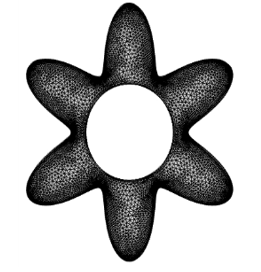Figure 2. Mesh of the present work, N=6, λ=4

To approach the results of the code, verification with the previous research Kim et. al.,  studied the natural convection inside the cold square enclosure having inner hot circular cylinder at different vertical locations and Shavic et. al. . The comparison showed a good confidence results for fluid flow, heat transfer and entropy generation which represent, by stream function, isotherms, entropy generation due to fluid flow and heat transfer and total entropy generation as in Figure 3a-b.

Table 1. Grid sensitivity, Ra =106, Amp=2, N=6

 Predefined mesh size $N u_{a v e}$ for $R a$$=10^{6}$ Error percentage% Extremely coarse 8.6648 Extra coarse 8.6419 -0.2649 Coarser 8.6982 0.6 Coarse 8.737 0.444 Normal 8.8056 0.779 Fine 8.8692 0.717 Finer 8.9174 0.54 Extra fine 8.9321 0.1645 Extremely fine 8.9320 0.01

## 3a1.jpg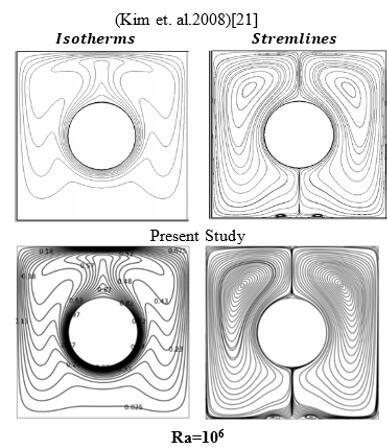## 3a2.jpg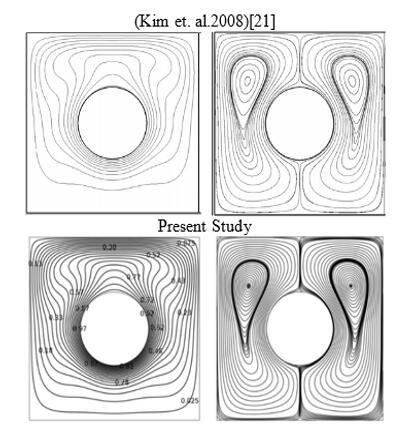3a). Comparison between  and present work for Ra=105, 106

## 3b.jpg## 3b2.jpg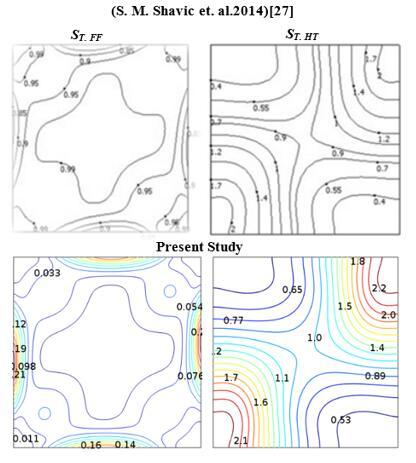## 3b3.jpg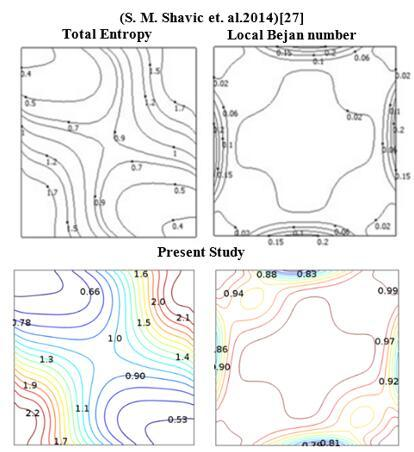3b). Comparison between (S. M. Shavic et. al., 2014)  and present work for Ra=103

Figure 3. Comparison between the present work and the other researchers

5. Results and Discussion

A numerical study has been achieved at the following values; Rayleigh number from 103 to 106 amplitude from 1 to 4. the effect of Rayleigh number on streamlines, isotherms, heat lines, and entropy generation, are shown in figures 4, 5, 6 7, 8, 9, and 10, respectively.

5.1 Flow field

Figure 4, depicts the shapes of streamlines function with amplitude λ=1, 2 and 4, and Ra=103 to 106. At Ra=103 and λ=1 and 2 and based on the nature of fluid circulation from the hot to the cold walls, the enclosure is occupied with two major irregular-symmetric vortexes beside another four secondary vortexes distributed at the core of every major vortex, two clockwise on the right and two anti-clockwise on the left. When the amplitude increased to 4 (the length of the outer cold wall increased), the resistance of the fluid circulation increased and the fluid flow damped from Ψmax.=3.62 to Ψmax.=2.3497, respectively.  Moreover, small secondary vortexes are seen at the upper and lower crests when Rayleigh number and the amplitude increased to Ra =104 and λ=1, respectively. In addition, it is noted that the buoyancy force increased and that led to shift the two secondary left and right vortexes to the lower half of the enclosure and federated with the other two vortexes at the upper half. The shifting and the federation processes formed two major, dominant, symmetrical and irregular shaped vortexes occupied both sides of the enclosure. However, at λ =2, a simple different behavior is noticed as compared to what was presented when Ra=103. Two secondary vortexes are observed at the upper crest. With further increasing in the amplitude λ=4, the previous four major vortexes occurred when Ra=103, are completely separated and distributed in the left and right sides. As well as, the vorticity of a couple of secondary vortexes at the upper zone of the enclosure showed a stronger dependence on the weakness of the lower vortexes than that seen in the previous results due to increase the buoyancy force. Hence, Ψmax. decreased from 22.14 to 13.3949. A significant increment in the buoyancy force is noticed at λ=1 and Rayleigh number increased from Ra=105 to 106. Due to that, the core of the two dominant vortexes explained previously elongated toward the top crest of the enclosure at Ra=105. It should be noted that the smooth and symmetrical curves of streamlines at Ra=105 begins to distort and showed asymmetric forms at highest Rayleigh number Ra=106 due to the presence of a strong buoyancy force. Obviously at λ=2 and Ra=105, the vorticity increased to 55.45 while it was 19.13 at Ra=104. In addition, the four large vortexes seen at Ra=104 compacted to two only. Besides that, another four secondary and weak vortexes accumulated at the upper crest of the enclosure, so that at Ra=106, the streamlines are disturbed only as vorticity increases to 83.71. With further increment of the amplitude λ=4, the lower half vortexes seen at Ra=104 started to be vanished and the streamlines deformity showed a clear conforming with increasing the buoyancy intensity particularly at Ra=106. In addition, Ψmax. reached to the highest value of 185.68 at the previous values of Rayleigh and amplitude. In general, it should be indicating that stream function value is clearly going up with increasing Rayleigh number

## 4.pngFigure 4. Streamlines for different Rayleigh number and amplitude

5.2 Temperature field

The isotherms are shown in Figure 5 for different values of Rayleigh numbers and amplitudes. The isotherms are presented patterns at Ra=103 and amplitudes of λ=1, 2 and, 4. It should be noted, at λ=2 and 4, the red color propagated away and around the inner hot wall consequential from surfaces approaching. Therefore, the isotherm lines clustered near the hot wall, and the pure conduction heat transfer is the dominated mode. With increasing Rayleigh number to 104 and λ=1, the convection eddies are developing producing a symmetrical flame ring around the inner wall. Further increasing of the amplitude λ=2 allows the surface to approach and that deforms the isothermal lines, but still symmetrical about the vertical axis. With further increasing in the amplitude λ=4, the thermal boundary layer clustered and reposed around the inner circular wall. The conduction heat transfer mode dominated as well.

The convection heat transfer mode is almost dominated at Ra =105 and 106 and λ=1, 2, and 4. It should be noted that the effective half of heat transfer processes is the upper section, which depends on the fact that says, the active heat transport is mainly occur based on the location of the hot surface with respect to cold one. Simultaneously, at the lowest crest, the isotherm lines are either parallel or nonexistent, i.e. weak temperature gradient at this zone. That makes it easy to understand why the conduction heat transfer mode is the dominant at this zone. Finally, after scrutinizing the isotherms disturb, it is noted that its characteristic of symmetry alternated due to turbulent fluid circulation at when Ra=106.

## 5.pngFigure 5. Isotherms for different Rayleigh number and amplitude

5.3 Heatlines field

Figure 6 indicates the heat lines between the inner cylinder and outer wavy cylinder for Ra=103 to 106 and the amplitude varies from λ=1 to 4. The positive and negative signs of $\Pi$  refer to the anti-clockwise (minimum) and clockwise (maximum) directions, respectively of the intensity and the fluid heat flow circulation.

## 6.png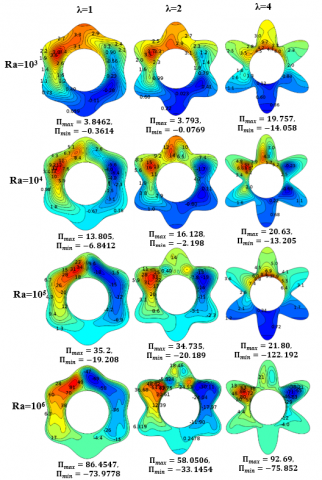Figure 6. Heatlines for different Rayleigh number and amplitude

For Ra=103 and λ=1, the conduction mode is the dominant as well as the lower circulation index seen Ψmax.=3.62 as shown in Figure 4. Two weak vortexes of heat lines are observed at the upper left and lower right of the enclosure. It should be noted that the heat lines connected between the inner to the outer walls of heat flow have the bushiest value and longest bath. Further, as λ increases to 2, the stream function is suppressed from Ψmax.=3.62 to 3.405 shown in, Figure 4 and the conduction heat dissipation near the inner wall of isotherms shown in Figure 5, are observed. Therefore, four weak vortexes of heat lines are shown synonymously with that of streamlines in Figure (4), and the heat lines value is dropped. As the amplitude increases to 4, the vorticity of heat lines almost disappeared, and the positive heatlines patterns increased from 3.8462 to 19.757. It was observed that the heat lines observed to be shortest in length. This refers to the biggest amount of conduction heat that transferred at this case where Πmax.=19.757 at Ra=103.

As Ra increases to 104 and λ=1, the circulation of fluid flow increases. Hence the convective energy transfer also increases from Πmax.=3.846 to 13.805, which is reflected on heat flow paths. It should be noted that when λ=2, the fluid heat flow circulations started to split and molded several heat flow vortexes. Then, at Ra=105 and 106, an important point to be noted, the heat lines joined together between the walls in a decreased trend and converted to two and large dominant heat flow vortexes. On the other hand, the heat flow circulations centers accumulated at the upper half of the enclosure, due to buoyancy force increasing, forming two large dominant fluid heat flow circulations.

A deep checking for the heat lines behavior at Ra=105 and 106 and λ=2 reveals that the fluid flow is damped. As a result for the approaching between the concave down of the external wall and the inner wall, large heat flow circulations is divided into several small circulations. The circulations distribution and its number are sensitive to the strength of the buoyancy force. As λ=4 and Ra=105, the fluid heat flow circulations disappeared and this case represents the perfect case to heat transfer with Πmin.=-122.192. However, at Ra= 106, the lifting buoyancy force is reactivated again and returned to be the dominant. Then the circulation of the heat flow appeared again at the upper half of the enclosure with a maximum value of Πmax.=86.4547. In the present work, one can conclude that the heat flow patterns are governed by the combined effects of streamlines and isotherms functions.

5.4 Entropy field

Figures 7-9 show the entropy generation due to heat transfer and fluid flow for Ra=103 to 106 and amplitude varies from λ=1 to 4. As mentioned previously, the total entropy generation for the presented study was coming from two secondary impressions of entropy generation as a result of heat transfer and fluid flow. It was noted from Figure 7, when λ=1 and 2 and Ra=103, the local entropy map due to heat transfer distributed uniformly filling the enclosure. As the Rayleigh number increases to 104, the buoyancy force enhanced and then the local entropy generation due to heat transfer started to dense gradually and became closer to the enclosure walls. That in turn, formed a thin layer at Ra=106 in the direction of the enclosure walls at Ra=106. However, at λ=4, the entropy generation maps crowded around the hot cylindrical wall. This was due to the convergence of the enclosure walls. Two important remarks should be reported here: the first is the entropy generation due to heat transfer and isotherms contours are homology. The second is that the values of the entropy generation increased with increasing Rayleigh number (such as from 3.55 to 313.54 when λ=1) due to increase the heat transfer rate. As well as, they increased with amplitude increasing (such as from 3.55 to 25 when Ra=103) as well as, Figure 8, demonstrated the local entropy generation due to fluid flow for different Rayleigh numbers and amplitudes. Concentration of entropy contours initiated at the left and right sides of the enclosure at Ra=103 and 104 for various amplitude. This was because of strong vorticity of fluid accumulated at left and right as shown in streamlines contours. As the Rayleigh number increased to 105 and 106, the buoyancy force enhanced, then the entropy lines scavenged to the upper half of the enclosure. Furthermore, the entropy generation showed its strongest values nearest the enclosure walls, while they are weak in the other regions. Similarly, the entropy generation due to fluid flow, increases with Rayleigh number and amplitude.

Figure 9 shows the total entropy generation for different Rayleigh numbers and amplitudes. It is noticed that at low Rayleigh number Ra=103 and the amplitude varies from λ =1 to 4, the total entropy generation contours showed similar behavior to their local entropy generation due to heat transfer because it is higher and more dominant than entropy generated by fluid flow. In contract, when Rayleigh number varied from Ra=104 to 106 and the amplitude varied from λ=1 to 4, the total entropy generation due to fluid flow is higher than the entropy generation due to heat transfer. Therefore, the total entropy generation contours have similar behavior to entropy generation due to fluid flow.

## 7.png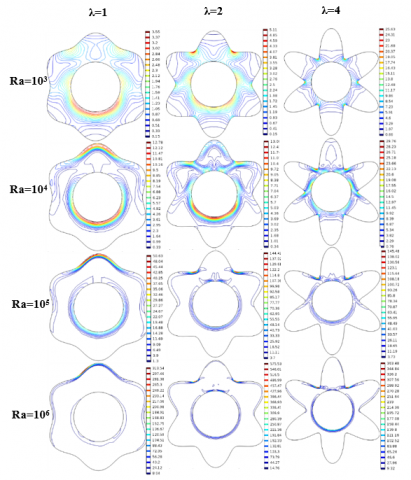Figure 7. Local entropy generation due to heat transfer for different Rayleigh number and amplitude (λ)

## 8.png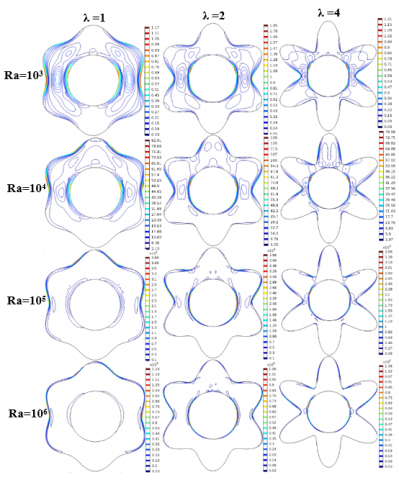Figure 8. Local entropy generation due to fluid flow for different Rayleigh number and amplitude

## 9.pngFigure 9. Total entropy generation for different Rayleigh number and amplitude

## 10.png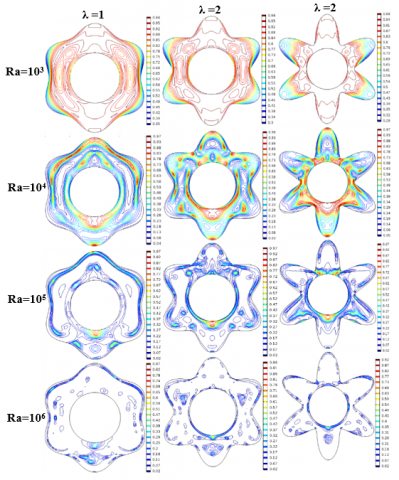Figure 10. Bejan Number for different Rayleigh number and amplitude

5.5 Bejan number

The values of Bejan number for different Rayleigh numbers and amplitudes are arranged in Figure 10. The distribution of Bejan number shows the regions with high fluid friction where Bejan number have the highest values. With increasing Rayleigh number, the effect of fluid flow increases and the Bejan number values decrease consequently, which illustrates the irreversibility of fluid friction effect and the domination of the entropy generation due to fluid flow. When Ra=103 and amplitude varies from λ=1 to 4, the Bejan number values stay unchanged, but for other values of Rayleigh numbers, the amplitude increases the reposition of the fluid circulation and reduces the fluid friction and Bejan number.

5.6 Local Nusselt number

Figure 11, illustrated the relation between the local Nusselt Number and the amplitude for different Rayleigh Number along the inner hot wall of the enclosure. In the beginning, as explained previously, the maximum local Nusselt Number is obtained at the gaps of convergent surfaces, and the minimum at the divergent, which forming the fluctuations. At low Ra=103, θ =0 to 180 i.e. lower half of the enclosure, Figure 11, as expected the local Nusselt Number having the highest fluctuation value, due to the reality of increasing of the effective area of the outer cold wall to heat transfer at λ =4. As Ra increases from 104 to 106, the convection heat and buoyancy force are increasing, thereby, the lifting force scavenge the flame to the upper half of the enclosure. Accordingly, the fluctuation of local Nusselt Number value at the lower half of the enclosure is damped for all values of Amplitude, seemed like a curved bundle especially at Ra =106. At low Ra =103, θ =180 to 360 i.e. upper half of the enclosure, Figure 11, the maximum fluctuation of λ=4 are continuous and didn’t almost affect. But with the increasing of Rayleigh Number from 104 to 106, the competition is released between the convection heat transfer flow effect and the increasing of the effective area of the cold wall by Amplitude increases. Thereafter, the fluctuation of the local Nusselt Number at λ=2 begins arises and revolt higher than the λ=4 and seems enclosed between θ=240 to 300 at Ra=106. Obviously, the value of the local Nusselt Number from θ=0 to 360, is increasing with increasing of Rayleigh Number for all values of Amplitude. As well as, the value of the local Nusselt Number at λ=1 seems didn’t effect dramatically with Rayleigh Number, started with weak fluctuation and finished with a form of the dewdrop.

## 11a.pngFigure 11. Local Nusselt number around Inner hot cylinder for different amplitude (λ) and Rayleigh number

Figure 12 represented the average Nusselt number with Rayleigh number for different amplitude, generally the average Nusselt number increased as the Rayleigh number increased due to enhancement of convection dominant. Consequently, the value of the local Nusselt Number at Ra=6*104 and λ=2 and 4, Figure 12, are intersected and inverted as a reflection of the above explanation.

## 12.png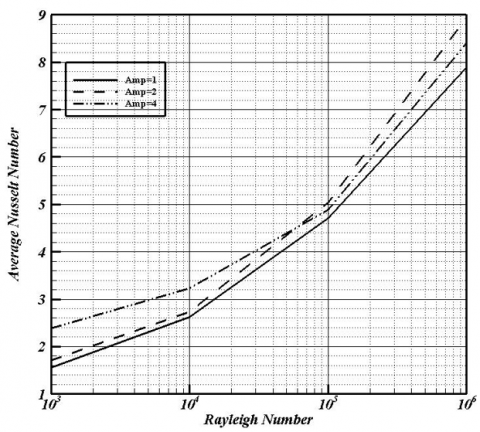Figure 12. Average Nusselt Number around Inner hot Cylinder Vs. Rayleigh Number at Different Amplitude (λ)

6. Conclusions

The laminar free convection in an enclosure formed by a hot inner circular cylinder and a cold sinusoidal outer cylinder at a different amplitude (λ=1,2,4) and Rayleigh number ranges (103-106), is diffracted numerically by finite element method. Galerkin technique is used to get the heatlines, streamlines, isotherms and entropy generation. The inner and outer cylinders are maintained at a constant temperature. Air is used as a working fluid with Prandtl number (0.71). It was denoted that the heat lines lengths become shortest and the heat flow circulation are disappeared as the amplitude increases. Hence, it can be said when the amplitude increases the perfect cases of heat transfer are occurred. But with respect to entropy generation, it was denoted at lower Rayleigh number the entropy generation is governed by heat transfer only as the amplitude increases. Vice versa, at highest Rayleigh number the entropy generation is governed by fluid flow only as the amplitude increases. Some conclusions can be drawn from the results of this work, such as:

(1) It is because of the fact that the enclosed path between the hot inner circular and cold outer corrugated enclosure walls is narrowest with the rise in amplitude, so that the fluid at these spaces is almost stagnant, higher rates of heat transfer are resulted.

(2) Generally, the absolute value of stream function enhanced with increasing Rayleigh number for all values of amplitudes.

(3) The fluid circulation is governed by two combined factors, the first one is the buoyancy force excited by increasing of Rayleigh number, so high fluid circulation occurred, and the second one, is flow damping due to amplitude increasing.

(4) The strongest fluid circulation is noticed at the upper half of the enclosure and returned to the presence of the hot wall under the cold one. Otherwise, the weakest, at the lower half, returned to the presence of the hot above the cold one.

(5) At a low Rayleigh number, the isothermal lines comply with the shape of the inner circular and outer corrugated walls, therefore the isothermal lines are concerned with the walls to refer that the conduction heat transfer are dominant.

(7) The sense of the heat lines is matching with the streamlines sense and more than of isotherms sense.

(8) That the increasing of amplitudes from λ=1 to λ=4 led to bend the streamlines, isotherms and heat lines.

(9) The maximum Nusselt number was noticed at the gaps of convergent surfaces at the upper of the enclosure half owing to the stagnation of fluid flow at these gaps.

(10) The local Nusselt number at Ra =103 and amplitude varies from λ=1 to λ=4 is fluctuated from θ =0o to 360o and recedes gradually from θ =240o to 300o with increasing of Rayleigh number to 106, as a result of convection.

(11) At a low Rayleigh number Ra=103, the total entropy generation is affected by the local entropy generation due to heat transfer, but at high Rayleigh number range (Ra=104 to 106), the total entropy is effected by local entropy generation due to fluid flow.

Nomenclature
 Cp Specific heat at constant pressure (KJ/kg.K) G Gravitational acceleration (m/s2) K Thermal conductivity (W/m.K) R Radius differences of inner and outer cylinder cavity (m) Ro Base circle (m) S Entropy P Dimensionless pressure P Pressure (Pa) Pr Prandtl number (νf/αf) Ra Rayleigh number $\boldsymbol{g} \boldsymbol{\beta}_{f} \boldsymbol{L}^{3} \boldsymbol{\Delta} \boldsymbol{T} / \boldsymbol{v}_{f} \boldsymbol{\alpha}_{f}$ T Temperature (K) Tc Temperature of the cold surface (K) Th Temperature of the hot surface (K) NuL Local Nusselt number on the hot inner cylinder $\mathrm{Nu}_{\text {ave }}$ Average Nusselt number hot inner circular cylinder U Dimensionless velocity component in x-direction u Velocity component in x-direction (m/s) V Dimensionless velocity component in y-direction v Velocity component in y-direction (m/s) X Dimensionless coordinate in horizontal direction x Cartesian coordinates in horizontal direction (m) Y Dimensionless coordinate in vertical direction y Cartesian coordinate in vertical direction (m) Gr Grashof number Greek symbols α Thermal diffusivity (m2/s) θ Dimensionless temperature (T-Tc/ΔT) $\Psi$ Dimensional stream function (m2/s) $\Psi$ Dimensionless stream function φ Angle of circular cylinder Φ irreversibility μ Dynamic viscosity (kg.s/m) ν Kinematic viscosity (μ /ρ)(Pa. s) β Volumetric coefficient of thermal expansion (K-1) ρ Density (kg/m3) Π Heat function Subscripts c Cold f Fluid (pure) h hot T total Abbreviations Min Minimum Amp. Amplitude (m) L local Max Maximum n Number of corrugations ave Average
References

 Sheikholeslami, M., Rokni, H.B. (2017). Influence of EFD viscosity on nanofluid forced convection in a cavity with sinusoidal wall. Journal of Molecular Liquids, 232: 390-395. https://doi.org/10.1016/j.molliq.2017.02.042

 Sheikholeslami, M., Rokni, H.B. (2017). Magnetohydrodynamic CuO–Water nanofluid in a porous complex-shaped enclosure. Journal of Thermal Science and Engineering Applications, 9(4): 041007. https://doi.org/10.1115/1.4035973

 Sheikholeslami, M., Shehzad, S.A. (2018). CVFEM simulation for nanofluid migration in a porous medium using Darcy model. International Journal of Heat and Mass Transfer, 122: 1264-1271. https://doi.org/10.1016/j.ijheatmasstransfer.2018.02.080

 Sheikholeslami, M., Shamlooei, M. (2017). Magnetic source influence on nanofluid flow in porous medium considering shape factor effect. Physics Letters A, 381(36): 3071-3078. https://doi.org/10.1016/j.physleta.2017.07.028

 Sheikholeslami, M., Oztop, H.F. (2017). MHD free convection of nanofluid in a cavity with sinusoidal walls by using CVFEM. Chinese Journal of Physics, 55(6): 2291-2304. https://doi.org/10.1016/j.cjph.2017.09.006

 Sheikholeslami, M., Ganji, D.D. (2017). Numerical approach for magnetic nanofluid flow in a porous cavity using CuO nanoparticles. Materials & Design, 120: 382-393. https://doi.org/10.1016/j.matdes.2017.02.039

 Sheikholeslami, M., Gorji-Bandpy, M., Pop, I., Soleimani, S. (2013). Numerical study of natural convection between a circular enclosure and a sinusoidal cylinder using control volume based finite element method. International Journal of Thermal Sciences, 72: 147-158. https://doi.org/10.1016/j.ijthermalsci.2013.05.004

 Sheikholeslami, M., Ellahi, R. (2015). Electrohydrodynamic nanofluid hydrothermal treatment in an enclosure with sinusoidal upper wall. Applied Sciences, 5(3): 294-306. https://doi.org/10.3390/app5030294

 Sheikholeslami, M., Hashim, I., Soleimani, S. (2013). Numerical investigation of the effect of magnetic field on natural convection in a curved-shape enclosure. Mathematical Problems in Engineering, 2013. http://dx.doi.org/10.1155/2013/83/725

 Tang, W., Hatami, M., Zhou, J., Jing, D. (2017). Natural convection heat transfer in a nanofluid-filled cavity with double sinusoidal wavy walls of various phase deviations. International Journal of Heat and Mass Transfer, 115: 430-440. https://doi.org/10.1016/j.ijheatmasstransfer.2017.07.057

 Sheremet, M.A., Pop, I., Roşca, N.C. (2016). Magnetic field effect on the unsteady natural convection in a wavy-walled cavity filled with a nanofluid: Buongiorno's mathematical model. Journal of the Taiwan Institute of Chemical Engineers, 61: 211-222. https://doi.org/10.1016/j.jtice.2015.12.015

 Yousaf, M., Usman, S. (2015). Natural convection heat transfer in a square cavity with sinusoidal roughness elements. International Journal of Heat and Mass Transfer, 90: 180-190. https://doi.org/10.1016/j.ijheatmasstransfer.2015.06.049

 Sheremet, M.A., Pop, I. (2015). Natural convection in a wavy porous cavity with sinusoidal temperature distributions on both side walls filled with a nanofluid: Buongiorno's mathematical model. Journal of Heat Transfer, 137(7): 072601. https://doi.org/10.1115/1.4029816

 Nabavizadeh, S.A., Talebi, S., Sefid, M., Nourmohammadzadeh, M. (2012). Natural convection in a square cavity containing a sinusoidal cylinder. International Journal of Thermal Sciences, 51: 112-120. https://doi.org/10.1016/j.ijthermalsci.2011.08.021

 Öztop, H.F., Sakhrieh, A., Abu-Nada, E., Al-Salem, K. (2017). Mixed convection of MHD flow in nanofluid filled and partially heated wavy walled lid-driven enclosure. International Communications in Heat and Mass Transfer, 86: 42-51. https://doi.org/10.1016/j.icheatmasstransfer.2017.05.011

 Oztop, H.F., Abu-Nada, E., Varol, Y., Chamkha, A. (2011). Natural convection in wavy enclosures with volumetric heat sources. International Journal of Thermal Sciences, 50(4): 502-514. https://doi.org/10.1016/j.ijthermalsci.2010.10.015

 Sompong, P., Witayangkurn, S. (2012). Simulation of natural convection in a complicated enclosure with two wavy vertical walls. Applied Mathematical Sciences, 6(57-60): 2833-2842.

 Nasrin, R. (2011). Mixed magnetoconvection in a lid-driven cavity with a sinusoidal wavy wall and a central heat conducting body. Journal of Naval Architecture and Marine Engineering, 8(1): 13-24. https://doi.org/10.3329/jname.v8i1.6793

 Khanafer, K., Al-Azmi, B., Marafie, A., Pop, I. (2009). Non-Darcian effects on natural convection heat transfer in a wavy porous enclosure. International Journal of Heat and Mass Transfer, 52(7-8): 1887-1896. https://doi.org/10.1016/j.ijheatmasstransfer.2008.08.040

 Mahmud, S., Das, P.K., Hyder, N., Islam, A.S. (2002). Free convection in an enclosure with vertical wavy walls. International Journal of Thermal Sciences, 41(5): 440-446. https://doi.org/10.1016/S1290-0729(02)01336-4

 Kim, B.S., Lee, D.S., Ha, M.Y., Yoon, H.S. (2008). A numerical study of natural convection in a square enclosure with a circular cylinder at different vertical locations. International Journal of Heat and Mass Transfer, 51(7-8): 1888-1906. https://doi.org/10.1016/j.ijheatmasstransfer.2007.06.033

 Hussain, S.H. (2016). Analysis of heatlines and entropy generation during double-diffusive MHD natural convection within a tilted sinusoidal corrugated porous enclosure. Engineering Science and Technology, an International Journal, 19(2): 926-945. https://doi.org/10.1016/j.jestch.2015.12.001

 Metrod, J., AL-Naffiey, H.K., Ali, F.H. (2010).Thermal analysis of laminar free convection in horizontal annulus with triangle radial fins attached to inner cyliner. Journal of Babylon University, 18(3): 13-24.

 Al-Amir, Q.R., Alinnawi, F.H.A., Hamzah, H.K. (2017). Effect of wavy wall location on the natural convection in an enclosure containing a concentric heated circular cylinder. Iraqi Journal of Mechanical and Material Engineering, 17(2): 291-308.

 Basak, T., Aravind, G., Roy, S., Balakrishnan, A.R. (2010). Heatline analysis of heat recovery and thermal transport in materials confined within triangular cavities. International Journal of Heat and Mass Transfer, 53(19-20): 3615-3628. https://doi.org/10.1016/j.ijheatmasstransfer.2010.03.028

 Natarajan, E., Basak, T., Roy, S. (2008). Natural convection flows in a trapezoidal enclosure with uniform and non-uniform heating of bottom wall. International Journal of Heat and Mass Transfer, 51(3-4): 747-756. https://doi.org/10.1016/j.ijheatmasstransfer.2007.04.027

 Shavik, S.M., Hassan, M.N., Morshed, A.M., Islam, M.Q. (2014). Natural convection and entropy generation in a square inclined cavity with differentially heated vertical walls. Procedia Engineering, 90: 557-562. https://doi.org/10.1016/j.proeng.2014.11.772

 Rahimi, A., Kasaeipoor, A., Malekshah, E.H. (2017). Natural convection analysis by entropy generation and heatline visualization using lattice Boltzmann method in nanofluid filled cavity included with internal heaters-Empirical thermo-physical properties. International Journal of Mechanical Sciences, 133: 199-216. https://doi.org/10.1016/j.ijmecsci.2017.08.044

 Rahimi, A., Kasaeipoor, A., Malekshah, E.H., Amiri, A. (2018). Natural convection analysis employing entropy generation and heatline visualization in a hollow L-shaped cavity filled with nanofluid using lattice Boltzmann method-experimental thermo-physical properties. Physica E: Low-Dimensional Systems and Nanostructures, 97: 82-97. https://doi.org/10.1016/j.physe.2017.10.004

 Hussein, A.K., Lioua, K., Chand, R., Sivasankaran, S., Nikbakhti, R., Li, D., Habib, B.A. (2016). Three-dimensional unsteady natural convection and entropy generation in an inclined cubical trapezoidal cavity with an isothermal bottom wall. Alexandria Engineering Journal, 55(2): 741-755. https://doi.org/10.1016/j.aej.2016.01.004

 Ghasemi, K., Siavashi, M. (2017). Lattice Boltzmann numerical simulation and entropy generation analysis of natural convection of nanofluid in a porous cavity with different linear temperature distributions on side walls. Journal of Molecular Liquids, 233: 415-430. https://doi.org/10.1016/j.molliq.2017.03.016

 Alsabery, A., Ishak, M., Chamkha, A., Hashim, I. (2018). Entropy generation analysis and natural convection in a nanofluid-filled square cavity with a concentric solid insert and different temperature distributions. Entropy, 20(5): 336. https://doi.org/10.3390/e20050336

 Abdulkadhim, A., Hamzah, H.K., Abed, M.A., Ali, H.F. (2017). Numerical study of entropy generation and natural convection heat transfer in trapezoidal enclosure with a thin baffle attached to inner wall using liquid nanofluid. In Annales de Chimie Science des Materiaux, 25(1-2): 7-28.

 Roslan, R., Saleh, H., Hashim, I., Bataineh, A.S. (2014). Natural convection in an enclosure containing a sinusoidally heated cylindrical source. International Journal of Heat and Mass Transfer, 70: 119-127. https://doi.org/10.1016/j.ijheatmasstransfer.2013.10.011

 Bouhalleb, M., Abbassi, H. (2016). Time response of natural convection of nanofluid CuO-H2O in enclosure submitted to a sinusoidal thermal boundary condition. Journal of Applied Fluid Mechanics, 9(3): 1157-1166.

 Alsabery, A.I., Chamkha, A.J., Saleh, H., Hashim, I., Chanane, B. (2017). Effects of finite wall thickness and sinusoidal heating on convection in nanofluid-saturated local thermal non-equilibrium porous cavity. Physica A: Statistical Mechanics and its Applications, 470: 20-38. https://doi.org/10.1016/j.physa.2016.11.107

 Zainuddin, N., Bakar, N.A., Karimipour, A., Roslan, R. (2006). Effect of sinusoidally heating on mixed convection in square cavity filled with a porous medium. ARPN J Eng Appl Sci, 12(7): 2351-7.

 Karim, A., Billah, M.M., Newton, M.T., Rahman, M.M. (2017). Influence of the periodicity of sinusoidal boundary condition on the unsteady mixed convection within a square enclosure using an Ag–water nanofluid. Energies, 10(12): 2167. https://doi.org/10.3390/en10122167

 Dutta, S., Biswas, A.K., Pati, S. (2018). Natural convection heat transfer and entropy generation inside porous quadrantal enclosure with nonisothermal heating at the bottom wall. Numerical Heat Transfer, Part A: Applications, 73(4): 222-240. https://doi.org/10.1080/10407782.2018.1423773

 Laouer, Djeghiour, R. (2019). Lattic Boltzmann simulation of magnetohydrodynamic free convection in a square enclosure with non-uniform heating of the bottom wall. Journal of Advanced Research in Fluid Mechanics and Thermal Sciences, 59: 13-28.